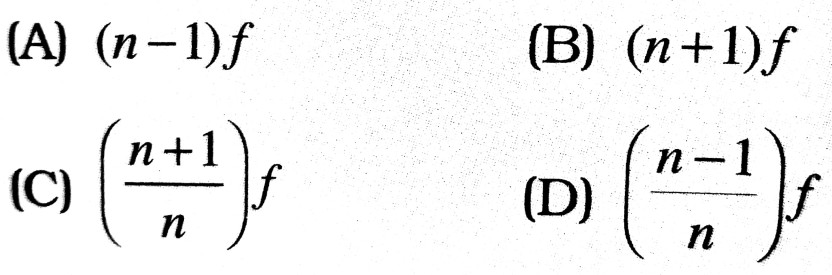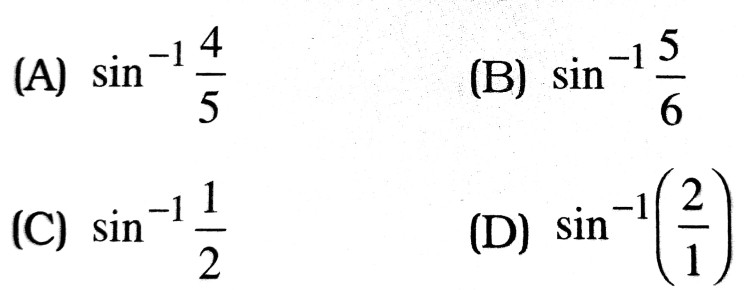1. Which of the following is not a property of light ?

(A) It can travel through vacuum
(B) It has a finite speed
(C) It involve transportation energy
(D) It requires a material medium for its propagation

2. Two points P and Q are situated at the same distance from a source of light but on opposite sides. The plane difference between the light waves passing through Pand I will be :

(A) n
(B) 2n
(C) π/2
(D) zero

3. The phase difference between the electric and the magnetic field vectors in electromagnetic waves is :

(A) π/4
(B) π/2
(C) π
(D) zero

4. A ray of light travelling in air is incident on a glass slab. The ray gets partly reflected and partly refracted. The phase difference between the reflected and the refracted waves is :

(A) π/2
(B) π/4
(C) π
(D) zero

5. Which of the following cannot be polarised ?

(B) transverse waves
(C) sound waves
(D) X-rays

6. Light year is the unit of:

(A) distance
(B) time
(C) intensity of light
(D) None of these

7. If a mirror is approaching you at a speed of 10 ms-1, the speed with which your image approach you is :

(A) 10 ms-1
(B) 5 ms-1
(C) 20 ms-1
(D) 15 ms-1

8. The refractive indices (R.I.) of glass and water with respect to air are 3/2 and 4/3 respectively. The R.I. of glass w.r. to water is :

(A) 8/9
(B) 9/8
(C) 7/6
(D) 2

9. The angle of minimum deviation for an equilateral glass prism is 30°. Refractive index of the prism is :

(A) 1/√2
(B) √3
(C) 1/3
(D) can’t be determined

10. A beam of light is incident at 60° to a plane surface. The reflected and refracted rays are perpendicular to each other. What is the R.I. of the surface :

(A) 1/√3
(B) √3
(C) 1/3
(D) 3

11. A concave mirror of focal length f produces an image n times the size of the object. If the image is real then the distance of the object is :12. A thin glass ( RI = 3/2 ) lens has optical power of – 5D in air. Its optical power in a liquid medium with RI = 1.6 will be :

(A) – 2.5 D
(B) 25 D
(C) – 1D
(D) 0.625

13. What happens to the interference pattern the two slits s1 and s2 in Young’s double experiment are illuminated by two independent but identical sources ?

(A) The intensity of the bright fringes doubled
(B) The intensity of the bright fringes becomes four times
(C) Two sets of interference fringes overlap
(D) No interference pattern is observed

14. What is the reason for your answer to the above question ?

(A) The two sources do not emit light of the same wavelength
(B) The two sources emit waves which travel with different speeds
(C) The two sources emit light waves of different amplitudes
(D) There is not constant phase difference between the waves emitted by the two sources

15. A single slit diffraction pattern is obtained using a beam of red light. What happened the red light is replaced by the blue light ?

(A) There is no change in diffraction pattern
(B) Diffraction fringes become narrower and crowded
(D) Diffraction fringes become broader and farther apart
(D) The diffraction pattern disappear

16. When a polaroid is rotated, the intensity of light varies but never reduces to zero. It shows that the incident light is :

(A) unpolarised
(B) completely plane polarised
(C) partially plane polarised
(D) None of the above

17. Two lens of power -15D and + 5D are placed in contact co-axially. The focal length of equivalent lens is :

(A) – 10 cm
(B) – 20 cm
(C) + 10 cm
(D) None

18. Refractive index of water and glass are 4/3 and 5/3. A light ray is going to water from glass. Then, its critical angle will be :19. The air bubble inside water shine due to :

(A) Reflection
(B) Refraction
(C) T.I.R.
(D) None of these

20. Far point of a myopic eye is 40 cm. The dioptic power of a corrective lens is : .

(A) 40D
(B) – 4D
(C) – 2.5 D
(D) – 0.25 diopter

21. Two thin lenses of focal lengths 20 cm and 25 cm are placed in contact. The effective power of the combination is :

(A) 45 D
(B) 9 D
(C) 1/9
(D) 6 D

22. A blue cross on the white background is illuminated with white light and is observed through red filter. What is seen ?

(A) A red cross on a black background
(B) A blue cross on a red background
(C) A red cross on a blue background
(D) A black cross on a red background

23. The angle of deviation for a prism is greatest for :

(A) violet
(B) red
(C) orange
(D) yellow

24. A double convex air bubble in water behaves as :

(A) Convergent lens
(B) divergent lens
(C) plane slab
(D) concave mirror

25. The focal length of a plane mirror of height 12 cm is :

(A) infinite
(B) 12 cm
(C) 6 cm
(D) 24 cm

26. If there were no atmosphere, the length of day on the earth will :

(A) decrease
(B) increase
(C) remain the same
(D) depends on the season of the year

27. When a ray of white light enters from air into a glass dense, it undergoes a change in :

(A) frequency only
(B) speed only
(C) Both (A) and (B)
(D) Wavelength and speed

28. Aray incident at 15° om one refracting surface of a prism of angle 60° suffers a deviation 55°. What is the angle of emergence ?

(A) 95°
(B) 45°
(C) 30°
(D) None of these

29. The intensity of light emerging from the two slits, in Young’s experiment is in the ratio 1 : 4. The ratio of the intensity of the minimum to that of the consecutive maximum will be:

(A) 1:4
(B) 1:9
(C) 1:16
(D) 2:3

30. What should be the refractive index of a completely transparent medium for it to be visible in vacuum ?

(A) 1
(B) less than 1
(C) greater than 1
(D) none of the above

31. The geometrical path of a ray of light in a medium of refractive index 2 is 8, then the optical path (in units of length) is :

(A) 4
(B) 8
(C) 16
(D) None of these

32. The time taken by light to travel a distance of 3 x 10-2 m is :

(A) 10-8 s
(B) 108 s
(C) 10-10 s
(D) 1010 s

33. The bending of a beam around comers of an obstacle is called :

(A) reflection
(B) refraction
(C) diffraction
(D) interference

34. Half period zones are so termed because, the path difference between two consecutive zones is :

(A) λ/4
(B) λ/2
(C) λ
(D) zero

35. A circular obstacle is placed 2m away from a point source c monochromatic light of λ = 5000 Å. The radius of the obstacle tha covers the first zone completely is :

(A) 2 mm
(B) 1.5 mm
(C) 1 mm
(D) 0.5 mm

36. Compared to blue light, the red light diffraction pattern spreads :

(A) less
(B) same
(C) more
(D) nothing can be said